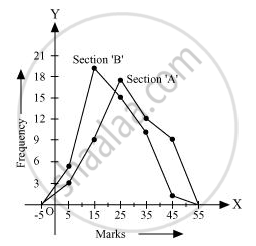# The Following Table Gives the Distribution of Students of Two Sections According to the Mark Obtained by Them - Mathematics

The following table gives the distribution of students of two sections according to the mark obtained by them:-

 Section A Section B Marks Frequency Marks Frequency 0 - 10 3 0 - 10 5 10 - 20 9 10 - 20 19 20 - 30 17 20 - 30 15 30 - 40 12 30 - 40 10 40 - 50 9 40 - 50 1

Represent the marks of the students of both the sections on the same graph by two frequency polygons. From the two polygons compare the performance of the two sections.

#### Solution

We can find the class marks of the given class intervals by using the following formula.

"Class mark"="Upper class limit + Lower class limit"/2

 Section A Section B Marks Class marks Frequency Marks Class marks Frequency 0 − 10 5 3 0 − 10 5 5 10 − 20 15 9 10 − 20 15 19 20 − 30 25 17 20 − 30 25 15 30 − 40 35 12 30 − 40 35 10 40 − 50 45 9 40 − 50 45 1

Taking class marks on x-axis and frequency on y-axis and choosing an appropriate scale (1 unit = 3 for y-axis), the frequency polygon can be drawn as follows.It can be observed that the performance of students of section ‘A’ is better than the students of section ‘B’ in terms of good marks.

Concept: Graphical Representation of Data
Is there an error in this question or solution?
Chapter 14: Statistics - Exercise 14.3 [Page 260]

#### APPEARS IN

NCERT Class 9 Maths
Chapter 14 Statistics
Exercise 14.3 | Q 6 | Page 260

Share